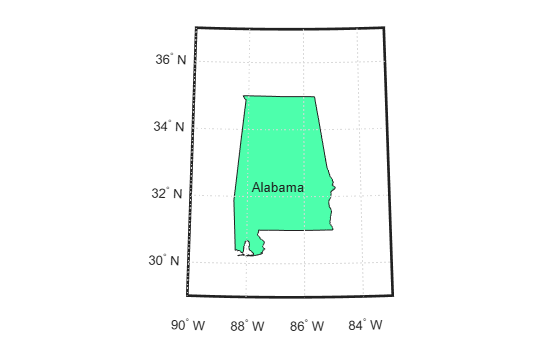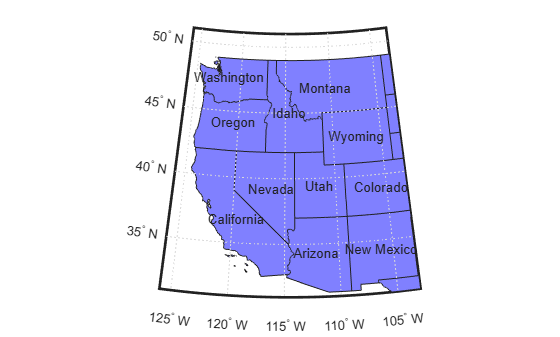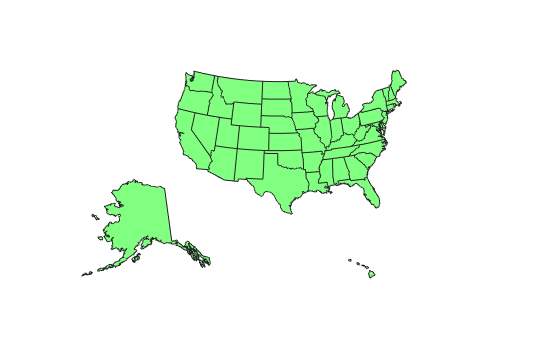# usamap

Construct map axes for United States of America

## Syntax

``usamap state``
``usamap(state)``
``usamap 'conus'``
``usamap('conus')``
``usamap``
``usamap(latlim,lonlim)``
``usamap(Z,R)``
``h = usamap(___)``
``h = usamap('all')``

## Description

example

````usamap state` and`usamap(state)` create an empty map axes with a Lambert Conformal Conic projection and map limits covering a U.S. state or group of states specified by `state`. The map axes is created in the current axes and the axis limits are set tight around the map frame.```
````usamap 'conus'` and`usamap('conus')` create an empty map axes for the conterminous 48 states (that is, all states excluding Alaska and Hawaii).```

example

````usamap` with no arguments presents a menu from which you can select a single state, the District of Columbia, the conterminous 48 states, or all states. ```
````usamap(latlim,lonlim)` creates an empty Lambert Conformal map axes for a region of the U.S. defined by its latitude and longitude limits in degrees.```
````usamap(Z,R)` derives the map limits from the extent of a regular data grid, `Z`, georeferenced by `R`.```
````h = usamap(___)` returns the handle of the map axes.```

example

````h = usamap('all')` constructs three empty map axes, inset within a single figure, for the conterminous states, Alaska, and Hawaii, with a spherical Earth model and other projection parameters suggested by the U.S. Geological Survey. The maps in the three axes are shown at approximately the same scale. The handles for the three map axes are returned in `h`.`usamap('allequal')` is the same as `usamap('all')`, but usage of `'allequal'` will be removed in a future release.```

## Examples

collapse all

Make a map of the state of Alabama only.

```figure usamap("Alabama") states = readgeotable("usastatehi.shp"); row = states.Name == "Alabama"; alabama = states(row,:); geoshow(alabama,"FaceColor",[0.3 1.0, 0.675])```

Label the state by adding text.

```textm(alabama.LabelLat,alabama.LabelLon,alabama.Name, ... "HorizontalAlignment","center")```Create a map of a contiguous landmass that contains California and Montana.

```figure ax = usamap({'CA','MT'}); set(ax,'Visible','off') states = readgeotable('usastatehi.shp'); geoshow(states, 'FaceColor', [0.5 0.5 1])```

Add labels to the states that are within the map limits.

```latlim = getm(ax,'MapLatLimit'); lonlim = getm(ax,'MapLonLimit'); lat = states.LabelLat; lon = states.LabelLon; tf = ingeoquad(lat,lon,latlim,lonlim); textm(lat(tf),lon(tf),states.Name(tf), ... 'HorizontalAlignment','center')```Map the conterminous United States. Color each state using a random, muted color.

```figure usamap("conus"); states = readgeotable("usastatelo.shp"); rows = states.Name ~= "Alaska" & states.Name ~= "Hawaii"; states = states(rows,:); h = height(states); faceColors = makesymbolspec("Polygon",... {'INDEX',[1 h],'FaceColor',polcmap(h)}); geoshow(states,"DisplayType","polygon","SymbolSpec",faceColors)```

Set optional display settings.

```framem off gridm off mlabel off plabel off```Read a shapefile, containing polygon shapes for each of the US states and the District of Columbia, into a geospatial table. Find the table rows for the conterminous USA, Alaska, and Hawaii.

```states = readgeotable("usastatelo.shp"); rowConus = states.Name ~= "Hawaii" & states.Name ~= "Alaska"; rowAlaska = states.Name == "Alaska"; rowHawaii = states.Name == "Hawaii";```

Display each of the three regions on separate axes.

```figure ax = usamap("all"); set(ax,"Visible","off") stateColor = [0.5 1 0.5]; geoshow(ax(1),states(rowConus,:), "FaceColor",stateColor) geoshow(ax(2),states(rowAlaska,:),"FaceColor",stateColor) geoshow(ax(3),states(rowHawaii,:),"FaceColor",stateColor)```

Hide the frame.

```for k = 1:3 setm(ax(k),"Frame","off","Grid","off",... "ParallelLabel","off","MeridianLabel","off") end```## Input Arguments

collapse all

State to display, specified as a string scalar, string array, character vector or cell array of character vectors. Permissible values include names of states, standard two-letter U.S. Postal Service abbreviations for states, and `'District of Columbia'`.

Example: `usamap({'Maine','Florida'})` sets the map limits to cover the region spanning from Maine to Florida.

Latitude limits, specified as a two-element vector of the form `[southern_limit northern_limit]`.

Longitude limits, specified as a two-element vector of the form `[western_limit eastern_limit]`.

Data grid, specified as an M-by-N array. `Z` is a regular data grid associated with a geographic reference `R`.

Geographic reference, specified as one of the following.

TypeDescription
Geographic raster reference object

`GeographicCellsReference` or `GeographicPostingsReference` geographic raster reference object. The `RasterSize` property must be consistent with the size of the data grid, `size(Z)`.

Vector

1-by-3 numeric vector with elements:

`[cells/degree northern_latitude_limit western_longitude_limit]`
Matrix

3-by-2 numeric matrix that transforms raster row and column indices to or from geographic coordinates according to:

`[lon lat] = [row col 1] * R`

`R` defines a (non-rotational, non-skewed) relationship in which each column of the data grid falls along a meridian and each row falls along a parallel.

## Output Arguments

collapse all

Handle of the map axes, returned as a handle object.

If you use the syntax ```h = usamap('all')```, then `h` is array of handle objects. `h(1)` is for the conterminous states, `h(2)` is for Alaska, and `h(3)` is for Hawaii.

## Tips

• All axes created with `usamap` are initialized with a spherical Earth model having a radius of 6,371,000 meters.

• In some cases, `usamap` uses `tightmap` to adjust the axis limits tight around the map. If you change the projection, or just want more white space around the map frame, use `tightmap` again or `axis auto`.

• `axes(h(n))`, where `n = 1`, `2`, or `3`, makes the desired axes current.

• `set(h,'Visible','on')` makes the axes visible.

• `axesscale(h(1))` resizes the axes containing Alaska and Hawaii to the same scale as the conterminous states.

## Version History

Introduced before R2006a